Next: Robot workspace analysis Up: Examples of applications of Previous: A robot control problem   Contents

Robot singularity analysis

In the robot presented in the previous sections we have seen that the inverse kinematic relates the the distances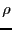between the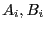to the position/orientation parameters of the platform X by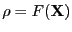. An important matrix is the jacobian matrix of this relation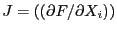. Indeed the forces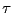in the legs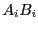are related to the forces/torques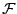applied on the platform by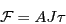where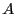is a matrix that is never singular. Hence for given forces/torques applied on the platform the force in each leg may be computed as a ratio whose denominator if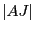. A configuration where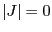is called a singularity of the robot. In this configuration the forces in the leg of the robot may go to infinity, causing a breakdown of the robot. Hence a very important practical problem is to be able to determine if there is a singularity within a given workspace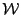of the robot.

Usually this workspace is defined in the following manner: a geometrical object that will include all possible location of a specific point of the platform and ranges for the parameters that describe the orientation of the platform.

The determinant of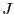can usually be expressed as a function of the position/orientation parameters. Interval analysis is appropriate to design an algorithm that check if there is a singularity within a given workspace. For that purpose we will take a point inside the workspace and calculate the value of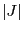at this point. Let us assume that this value is positive. A singularity will occur within the workspace if we are able to find another point in the workspace wherewill be negative.

We may assume that we are able to calculate a bounding box of the workspace and that we are able to design a test that allow to determine if a box for the position/orientation parameters is fully included in the workspace, is fully outside or part of it is inside and part of it is outside.

With that it is easy to design an algorithm that determine if the workspace includes a singularity (see  for more details).

First we use interval arithmetics to determine bounds for the value of. If the lower bound of the interval is positive is positive, then there is no singularity within the workspace. Otherwise we bisect the box. For the new boxes we first test if the box is inside the workspace: if one box is fully outside the workspace we discard it. If a box is not fully inside we bisect the box. For a box that is fully inside we compute the interval evaluation of. If the lower bound is positive we discard the box. If the upper bound is negative, then for any position/orientation defined by parameters within the box ranges the determinant will be negative: hence the workspace includes a singularity and we may stop the algorithm. If all the boxes in the list have been processed, then there is no singularity within the workspace.

Note that it is easy to generalize the algorithm so that uncertainties in the robot geometry are taken into account. A general version of this algorithm has been implemented using ALIAS and Maple and is able to deal with different robot architectures. Maple is used to compute the analytical form of. The ALIAS parser is used to perform the interval evaluation of the determinant.Next: Robot workspace analysis Up: Examples of applications of Previous: A robot control problem   Contents
Jean-Pierre Merlet 2012-12-20